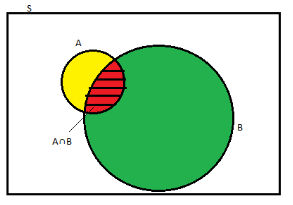# Conditional Probability and Conditional Probability Examples

Imagine a student who takes leave from school twice a week excluding Sunday. If it is known that he will be absent from school on Tuesday then what are the chances that he will also take a leave on Saturday in the same week? It is observed that in problems where the occurrence of one event affects the happening of the following event. These cases of probability are known as conditional probability.

Let us learn its definition mathematically,

## Conditional Probability Definition

The probability of occurrence of any event A when another event B in relation to A has already occurred is known as conditional probability. It is depicted by P(A|B).As depicted by above diagram, sample space is given by S and there are two events A and B. In a situation where event  B has already occurred, then our sample space S  naturally gets reduced to  B because now the chances of occurrence of an event will lie inside B.

As we have to figure out the chances of occurrence of event A, only portion common to both A and  B is enough to represent the probability of occurrence of A, when B has already occurred. Common portion of the events is depicted by the intersection of both the events  A and B i.e. A ∩ B.

This explains the concept of conditional probability problems i.e. occurrence of any event when another event in relation to has already occurred.

## Conditional Probability Formula

Mathematically this can be represented as,

 P(A|B) = N(A∩B)/N(B)

Where P(A|B) represents the probability of occurrence of A given B has occurred.

N(A ∩ B) is the number of elements common to both A and B.

N(B) is the number of elements in B and it cannot be equal to zero.

Let N represent the total number of elements in the sample space.

$$\begin{array}{l}\Rightarrow P(A|B)\end{array}$$
=
$$\begin{array}{l}\frac{\frac{N(A ∩ B)}{N}}{\frac{N(B)}{N}}\end{array}$$

Since N(A ∩ B)/N and N(B)/N denotes the ratio of the number of favourable outcomes to the total number of outcomes, therefore, it indicates the probability.

Therefore, N(A ∩ B)/N can be written as P(A ∩ B) and N(B)/N as P(B).

⇒ P(A|B) = P(A ∩ B)/P(B)

Therefore, P(A ∩ B) = P(B) P(A|B) if P(B) ≠ 0

= P(A) P(B|A) if P(A) ≠ 0

Similarly, the probability of occurrence of B when A has already occurred is given by,

P(B|A) = P(B ∩ A)/P(A)

To have a better insight let us practice some conditional probability examples.

### Conditional Probability Properties

Property 1: Let E and F be events of a sample space S of an experiment, then we have P(S|F) = P(F|F) = 1.

Property 2: f A and B are any two events of a sample space S and F is an event of S such that P(F) ≠ 0, then P((A ∪ B)|F) = P(A|F) + P(B|F) – P((A ∩ B)|F).

Property 3: P(A′|B) = 1 − P(A|B)

## Conditional Probability Example

Example:  Two dies are thrown simultaneously and the sum of the numbers obtained is found to be 7. What is the probability that the number 3 has appeared at least once?

Solution: The sample space S would consist of all the numbers possible by the combination of two dies. Therefore S consists of 6 × 6 i.e. 36 events.

Event A indicates the combination in which 3 has appeared at least once.

Event B indicates the combination of the numbers which sum up to 7.

A = {(3, 1), (3, 2), (3, 3)(3, 4)(3, 5)(3, 6)(1, 3)(2, 3)(4, 3)(5, 3)(6, 3)}

B = {(1, 6)(2, 5)(3, 4)(4, 3)(5, 2)(6, 1)}

P(A) = 11/36

P(B) = 6/36

A ∩ B = 2

P(A ∩ B) = 2/36

Applying the conditional probability formula we get,

$$\begin{array}{l} P(A|B)\end{array}$$
=
$$\begin{array}{l}\frac{P(A ∩ B)}{P(B)}\end{array}$$
=
$$\begin{array}{l} \frac{\frac{2}{36}}{\frac{6}{36}} \end{array}$$
=
$$\begin{array}{l}\frac{1}{3}\end{array}$$